# Mechanical and thermodynamic properties of Aβ42, Aβ40, and α-synuclein fibrils: a coarse-grained method to complement experimental studies

1Institute of Fundamental Technological Research, Polish Academy of Sciences, Pawińskiego 5B, 02-106 Warsaw, Poland
2Max Planck Institute for Polymer Research, Ackermannweg 10, 55128 Mainz, Germany
3Institute of Physics, Polish Academy of Sciences, Al. Lotników 32/46, 02-668 Warsaw, Poland

4Institute for Computational Science and Technology, Quang Trung Software City, Tan Chanh Hiep Ward, District 12, Ho Chi Minh City, Vietnam

1. Corresponding author email

Associate Editor: J. Frommer
Beilstein J. Nanotechnol. 2019, 10, 500–513. https://doi.org/10.3762/bjnano.10.51
Received 10 Oct 2018, Accepted 08 Feb 2019, Published 19 Feb 2019

• Full Research Paper

## Abstract

We perform molecular dynamics simulation on several relevant biological fibrils associated with neurodegenerative diseases such as Aβ40, Aβ42, and α-synuclein systems to obtain a molecular understanding and interpretation of nanomechanical characterization experiments. The computational method is versatile and addresses a new subarea within the mechanical characterization of heterogeneous soft materials. We investigate both the elastic and thermodynamic properties of the biological fibrils in order to substantiate experimental nanomechanical characterization techniques that are quickly developing and reaching dynamic imaging with video rate capabilities. The computational method qualitatively reproduces results of experiments with biological fibrils, validating its use in extrapolation to macroscopic material properties. Our computational techniques can be used for the co-design of new experiments aiming to unveil nanomechanical properties of biological fibrils from a point of view of molecular understanding. Our approach allows a comparison of diverse elastic properties based on different deformations , i.e., tensile (YL), shear (S), and indentation (YT) deformation. From our analysis, we find a significant elastic anisotropy between axial and transverse directions (i.e., YT > YL) for all systems. Interestingly, our results indicate a higher mechanostability of Aβ42 fibrils compared to Aβ40, suggesting a significant correlation between mechanical stability and aggregation propensity (rate) in amyloid systems. That is, the higher the mechanical stability the faster the fibril formation. Finally, we find that α-synuclein fibrils are thermally less stable than β-amyloid fibrils. We anticipate that our molecular-level analysis of the mechanical response under different deformation conditions for the range of fibrils considered here will provide significant insights for the experimental observations.

Keywords: β-amyloid; atomic force microscopy, mechanical deformation; molecular simulation; proteins; α-synuclein

## Introduction

All-atom molecular dynamics (MD) simulations have been employed to study the physical and chemical behaviour of the fundamental biomolecules of life (e.g., proteins , nucleic acids  and lipids ). Lipid membranes, viral capsids, and biological fibrils are common examples of large complexes that pose significant challenges for all-atom simulations. For example, the time scales of various biological processes are in the range from 10−6 to 10−3 s, and thus they are orders of magnitude larger than typical molecular motions (10−15 to 10−12 s) captured in all-atom MD. The length scales are similarly much smaller in all-atom simulations than it would be relevant for studying processes involving large conformational changes in large biological complexes. In the context of mechanical properties of various fibrils, for example, β-amyloids [4,5], cellulose  and collagen , all-atom models have been used to estimate the elastic moduli based on the response of the system, but mostly approximately. Still, molecular-level methods are necessary to understand the microscopic mechanisms of the mechanical response of biological fibrils. In this regard, coarse-grained (CG) models are suitable, because they remove several degrees of freedom of the system, which enables them to reach the experimental time and length scales that describe the relevant phenomena while maintaining a molecular-level description of the systems under consideration [8-11]. CG simulations are able to describe large structural changes in the context of fibril deformation, which would be otherwise impossible with all-atom models. In particular, the CG model can be used to infer the elastic parameter under ideal conditions, which is given by the Hertz model  and is valid for isotropic materials and as close as possible to the experimental conditions . While other sophisticated “Hertz-based models” [14,15] aim to study the elastic properties of anisotropic materials with high symmetries, e.g., crystals, such descriptions are not suitable for softer materials such as biological fibrils or polymers. Although biological matter is an example of an anisotropic material, it is not expected to follow a priori a simple Hertzian relationship given by FYTh3/2 (with YT being the transversal Young modulus and h being the indentation depth). If it actually follows this relationship, then the elastic modulus can be easily obtained from the slope of the curve. This approach can be used to test the experimental estimation of an elastic property. Most importantly, the mechanism of deformation that gives rise to the linear response can be characterized in the CG simulations. From the experimental point of view, there is a long-standing discussion in the atomic force microscopy (AFM) community whether Hertzian mechanics is applicable to all soft-matter samples explored with AFM. One of the basic assumptions of the Hertz model is that the indented object is a half-space and made out of a homogeneous material. However, at the nanoscale it is intrinsically difficult to measure pure and homogeneous materials, or perfectly mixed materials, with some exceptional cases, such as highly oriented pyrolytic graphite (HOPG), silica, and other “clean” surfaces, which are, however, very far away from biological systems. Moreover, by considering the indenter as a sphere, the anisotropies in the deformed material can be screened, since the measured deformation depends on the contact area, which will be the arc region that forms in contact with the sphere. In considering other shapes for the cantilever tip, such as conical or flat punch, the impact of the anisotropy is expected to be much higher . Nonetheless, to our knowledge the exact shape of the cantilever tip cannot be determined during experimental measurements. As a result, big discrepancies are found when comparing Young’s modulus values measured with macroscopic techniques and nanoscopic ones such as AFM. This is because a nanoscopic exploration of biological systems reaches molecular resolution and the measurements are in general very delicate due to the intrinsic properties of soft matter and the danger of damaging the samples . As a matter of fact, the employed reference model to study the mechanical response of biological fibrils during AFM nanoindentation has been also the Hertz model. Hence, we also use it as a reference for comparing the indentation values we obtained to the experimental ones, although we remark that our molecular modeling can adapt further anisotropic mechanical models, envisioned within force microscopy techniques.

Biological fibrils are well-known biomaterials of practical use. The related technological applications range from drug delivery  to structural scaffolds  in which the role of the fibril may be to immobilize small molecules such as enzymes ). The applications are motivated by the unique properties of fibrils, such as the spontaneous formation under certain conditions, the high mechanical stability (comparable to silk), and the ability to form ordered structures, albeit the monomeric units (proteins) of these fibrils are intrinsically disordered [21,22]. These are fundamental properties for applications in which the fragmentation of the material needs to be avoided, for example, syntheses, active processes (drug delivery) or responses to an external perturbation (change in temperature). To this end, the interplay between mechanical and thermodynamic properties will determine the overall behaviour of the fibrils, which depends on the arrangement of the individual amino-acid chains in these structures. Fibrils consisting of either 40-mer or 42-mer amyloid chains (it contains two additional hydrophobic amino acids) are particularly interesting. For example, Aβ40 typically assembles into two-fold or three-fold symmetries (see Figure 1), while the highest symmetry reported by experiments for Aβ42 fibrils is a two-fold symmetry, as in the case of α-synuclein (α-syn) fibrils [23,24]. Furthermore, the aggregation typically takes place 2.5-times faster in a solution of Aβ42 than in the case of Aβ40 [25,26]. Interestingly, the aggregation rate of fibril formation has been found to be highly correlated with the mechanical properties of the fibrils, namely, the mechanically more stable fibril is the one undergoing faster aggregation . While experimental observations have been derived from a small set of samples, our CG simulations can be used to validate these observations and study a larger set of fibrils.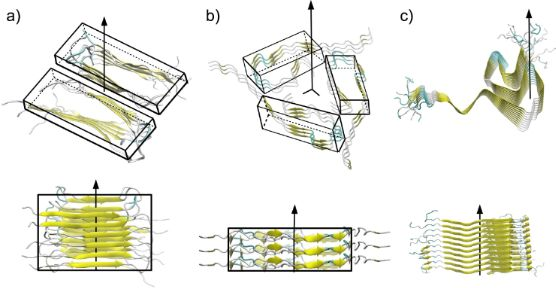Figure 1: Snapshots illustrating some of the biological fibrils used in our simulation. The main axis of symmetry and the secondary structure for each chain are indicated. (a) Aβ40 (PDB ID: 2LMO) with two-fold symmetry. (b) Aβ40 fibril (PDB ID: 2M4J) with three-fold symmetry. (c) α-syn fibril (PDB ID: 2N0A) with no symmetry. Rectangular boxes depict the local symmetry. Figure 1: Snapshots illustrating some of the biological fibrils used in our simulation. The main axis of symm... Jump to Figure 1

Typical length scales of biological fibrils are in the range from nanometers to micrometers. Hence, AFM that can be operated, for example, in static (contact) and dynamic modes, has been one of the main methods to study such systems [28,29]. On one hand, AFM in contact mode has been used to provoke the mechanical deformation of fibrils obtaining the Young’s modulus (here denoted as YT) [30-32]. On the other hand, the experimental determination of the tensile Young’s modulus (YL) is nontrivial at the nanoscale , due to the requirement of a different experimental setup, namely, the more involved sonification method . Moreover, the experimental calculation of the shear modulus (S) can be realised by suspending the fibril between two beams and pressing the free part against the indenter, which gives rise to the fibril bending modulus (Yb) that depends on both YT and S.

Our CG strategy can be used to extract and compare elastic properties in a systematic way. This significant advantage of CG simulations has motivated the current study, which employs MD simulation of a structure-based CG model [35-38] to investigate one α-synuclein and five β-amyloid fibrils of known experimental structure related to specific neurodegenerative diseases. Our simulation sheds light on the mechanical and thermodynamic properties of these fibrils by providing the microscopic picture required to explain the relevant phenomena. We achieve this by applying different types of deformation (e.g., tension, shearing and indentation) and analysing the intermolecular contacts between amino acids. Our simulations reveal significant differences in the mechanical behaviour between Aβ40 and Aβ42 and α-syn fibrils. Moreover, we find that the α-syn fibril is thermally less stable than the β-amyloid fibrils.

In the next section, we present details about our methodology. Then, we present our results and analysis, and in the last section we summarise our conclusions.

## Materials and Methods

For our studies, we have chosen three different Aβ40 fibrils with the PDB IDs 2LMO , 2M4J  and 2MVX  and two Aβ42 with the PDB IDs 5OQV , and 2NAO . The only available structure for α-syn is the one with PDB ID: 2N0A .

### The coarse-grained model

In our CG model, each amino acid is represented by a bead located at the Cα-atom position. The potential energy between the beads reads:

The first three terms on the right hand side of Equation 1 correspond to the harmonic pseudo-bond, bond angle and dihedral potentials. The values of the elastic constants were derived from all-atom simulations  and are Kr = 100 kcal/mol/Å2, Kθ = 45 kcal/mol/rad2 and= 5.0 kcal/mol/rad2. The choice of equilibrium values r0, θ0, andare based on two, three, and four α-C atoms, respectively, and are meant to favour the native geometry. The fourth term on the right-hand side of Equation 1 takes into account the non-bonded contact interactions, described by the Lennard-Jones potential. Here, we take εij to be uniform and equal to ε = 1.5 kcal/mol, which was also derived from all-atom simulations . Our approach has shown very good agreement with experimental data on stretching [46,47] and nanoindentation of biological fibrils, such as virus capsids  and β-amyloids . The strength of the repulsive non-native term, ε’, is set equal to ε. Our CG model takes into account native distances as in the case of a Gō-like model . Hence, the native contacts are determined by the overlap criterion . In practice, each heavy atom is assigned to a van der Waals radius, as proposed by Tsai and co-workers . A sphere with the radius enlarged by a factor of 1.24 is built around the atom. If two amino acids have heavy atoms with overlapping spheres, then we consider a native contact between those two Cα atoms. In Figure 2, we show the CG representation for some biological fibrils as well as their native interactions. These native contacts represent hydrogen bonds (HB), and hydrophobic and ionic bridges. Moreover, we consider contacts between amino acids in individual chains with sequential distance |ij| > 4. The parameters σij are given by rij0/2(1/6), where rij0 is the distance between two Cα atoms that form a native contact. The last term in Equation 1 simply describes the repulsion between non-native contacts. Here, we take rcut = 4 Å. Moreover, our terminology for the “contacts” in this manuscript, is as follows: i) intrachain contacts are considered those within a single chain, ii) interchain contacts are between two chains in a side-by-side configuration and iii) the intersheet contacts are found along the symmetry axis (see Figure 2). Below, we provide details on the different types of mechanical deformation, i.e., tensile, shear, and indentation processes.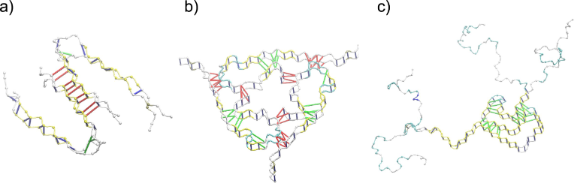Figure 2: Coarse-grained representation of the biological fibrils presented in Figure 1. Illustrated are the three types of “native contact” interactions considered in our study: i) intrachain contacts (green), ii) interchain contacts (red) iii) intersheet contacts (blue). Figure 2: Coarse-grained representation of the biological fibrils presented in Figure 1. Illustrated are the three ty... Jump to Figure 2

### Mechanical and thermodynamics characterization through a CG model

In our previous work , we have constructed a computational protocol for performing several types of mechanical deformation in silico (Figure 3). Such processes can be carried out at constant speed or force contact-modes. Here, we explore the former as it provides a dynamic picture of the whole process and it enables the characterisation of the mechanics during the early deformation stages. Moreover, we employ the CG simulation for the validation of the elastic theory. This is done by calculating the coefficient n in the indentation curves measuring the force as a function of hn. We found n = 3/2 in the linear regime, which corresponds to the Hertzian theory .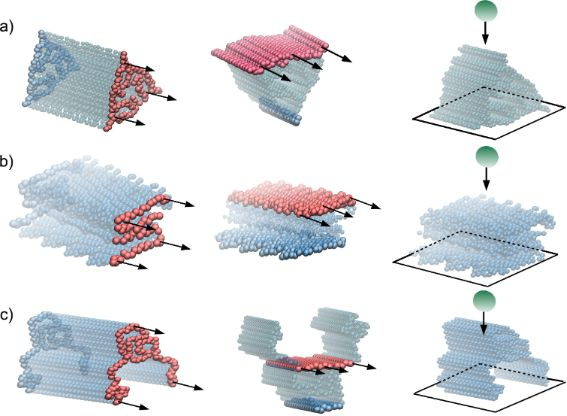Figure 3: For the cases of Figure 1, we present schematically each deformation process. The left panels show tensile, the middle panels shearing, and the right panels indentation processes. The set of Cα atoms anchored in each processes are shown in blue, the ones which are moving at a speed vpull are shown in red, and the indenter bead in green. Arrows indicate the direction of pulling. In the case of indentation, a potential z0−10 has been used to model the basis plane, where z0 is the distance between the plane and the CG beads. (a) Aβ40 (PDB ID: 2M4J), (b) Aβ40 (PDB ID: 2LMO) and (c) α-syn (PDB ID: 2NA0). Figure 3: For the cases of Figure 1, we present schematically each deformation process. The left panels show tensile,... Jump to Figure 3

#### Tensile deformation

The experimental calculation of the stress–strain data at the nanoscale can be done by using optical tweezers (OT) , AFM base-force spectroscopy , or by the design of sophisticated microelectromechanical systems (MEMS) . These techniques have been successfully used to predict the elastic properties of several biomolecules. However, OT are limited to applied loads below 0.1 nN and AFM has delicate calibration issues associated with a systematic deformation of samples of same length. In practice, all-atom simulation does not suffer from any of those drawbacks, but it cannot be used in biological systems. Instead, CG models are more suitable to achieve experimental length and time scales.

In practice, we set harmonic potentials to the furthest bottom and top particles of the protein. Then, we take values for the elastic constants equal to kbottom = 100 kcal/mol/Å and ktop = 0.1 kcal/mol/Å for the top part of the fibril. The top part is moving with pulling speed equal to vpull = 5 × 10−5 Å/ns. As a result of tensile deformation, the fibril stretches from a reference length (L0) to L, and the strain is given by. The stress is defined by the total force acting on the springs ktop divided by the cross-sectional area, A, of the sample. This area is calculated as follows : For a given set of Cartesian points, it determines the smallest convex polygon containing all the given points. Then, we monitor the elementary area of this polygon during the simulation . From the stress–strain plot one can derive the corresponding tensile Young’s modulus, YL.

#### Shear deformation

The experimental techniques employed before for the determination of YL are not applicable for the calculation of the shear modulus (S) at the nanoscale. Hence, an improved version of the single three-point bending technique was developed for the calculation of S . It combines a movement along the z-axis (perpendicular to the main fibril axis) with a continuous scanning motion along the main fibril axis. In this way, the slope dF/dz yields a better calculation of the bending modulus (Yb) and as a result a more accurate value of S. In comparison to its predecessor, this technique reduces the error in the value of S down to 12% in the case of collagen fibrils , but it still relies on the correct estimation of the fibril diameter. As above, the CG model helps to devise a protocol where simple shear planes can be applied on a set of atoms and the typical response allows, in a straightforward manner, for the calculation of S. In this case, we only couple the Cα-atoms from the top (ktop) and the bottom (kbottom) planes. The strain is defined by, where x is the displacement of the top plane and y is the height of the fibril (see Figure 3). The shear stress is calculated as the total force acting on the top plane divided by the area of the plane (see in Table 1 the reference Cα-atom used to define the top plane). From the stress–strain relation one can derive the corresponding shear Young’s modulus, S.

#### Indentation deformation

One of the empirical techniques used to estimate YT modulus is AFM nanoindentation. The wide range of applications of AFM technique span from biomolecules to single cells [31,56,57]. The AFM nanoindentation force–distance curves typically depend on the correct determination of the cantilever stiffness and only measurements of biological fibrils located at the centre of the fibril are considered. The former refers to the way that the indentation load is measured by the deflection of the AFM cantilever. The latter is an assumption of the semi-infinite half-space approximation. Once the AFM data is obtained, it requires interpretation by using a contact mechanics theory. There is no experiment at the nanoscale where the influence of the indenter could be neglected. Depending on the type of forces between the indenter and the biomaterial, we might describe the process by non-adhesive  or adhesive contact mechanics theories [58,59]. Here, we suggest our particle-based CG method as a tool for the modeling of the nanoindentation process. It is worth noting that we prevent any possible adhesion between the indenter and the fibril by placing a divergent interaction between the tip and the Cα atom, and hence other models [58,60] with such features are not considered. Moreover we chose the Young’s modulus of the indenter to be infinite and we define each system in the limit of the Hertzian theory . The indenter is a sphere with a radius of curvature Rind that moves towards the fibril with a speed vind. Then, the penetration or indentation depth (h) is measured from the first tip–particle interaction (or contact) and the associated indentation force (F) is calculated until the indenter stops being in contact with the fibril. From Hertz’s relation, it follows thatwhere ν is the Poisson coefficient, in this case equal to 0.5. This value corresponds to a homogeneous deformation in the xy-plane. From Hertz’s equation, we derive the transverse Young modulus, YT, in the linear regime of the Fh curve.

#### Thermodynamic characterization

The study of the thermal stability in the case of Aβ fibrils faces serious difficulties, stemming from the requirement for controlled in vitro preparation of samples with well-ordered Aβ40 or Aβ42 fibrils. In this regard, our CG simulation is an ideal protocol as it enables the calculation of the melting temperatures for well-ordered Aβ fibrils. To assess the thermal stability of the fibril, we compute the probability of finding the protein in the native state, P0, as a function of the temperature T. We define the temperature of thermodynamic stability, Tf (folding temperature in our model), for the case P0 = 1/2. To study the thermodynamic properties of the biological fibrils, we carried out overdamped Langevin dynamics simulations. The simulations were performed for 35 different temperatures, T, which were uniformly distributed in the range from 0.1ε/kB to 0.7ε/kB. Each simulation was 104τ long after running the systems for 103τ in order to reach equilibrium. In our studies, the unit of time, τ, is of the order of 1 ns. For this range of temperatures and time scales, we did not observe any dissociation or unfolding events for the fibrils. The deviation of the fibril structure from its native state was computed by means of the root mean square deviation (RMSD), which is defined as follows:

wheredenotes the positions of the Cα atoms in the native state andare the positions of the Cα atoms at time t after superimposing the native structure. After equilibration, RMSD fluctuates around an average value,, which is a function of the temperature T. In our case, the observed deviations from the native state in terms of RMSD are small at room temperature.

## Results and Discussion

### Tensile deformation

Our results for tensile deformation for all studied cases are illustrated in Figure 4. The initial length (L0) is measured after an equilibration of 100 τ. The cross-section area (A) for each system is monitored during the simulation and is shown as a function of the strain in the insets of Figure 4. The deviations are small compared to the mean value, especially in the case of β-amyloid fibrils. Hence, we calculated the stress using the average value of A. The values of the cross-section areas and the initial length for each fibril are listed in Table 1. The theoretical values of YL have been obtained for vpull = 0.0005 Å/τ are listed in Table 2, next to the experimental values for the sake of comparison. In our studies, the deformation is carried out along the main axis of symmetry (see Figure 1) for Aβ and α-syn fibrils. We find that the type of Aβ fibrils plays a more important role in the mechanical properties than the symmetry of each fibril. This becomes apparent by comparing the values of the tensile Young’s modulus of Aβ40 and Aβ42. Our discussion is based on the average values of YL. In the case of Aβ40, we obtain YL = 2.1 GPa, while for Aβ42 this value is 2.4 GPa. The value YL = 2.3 GPa in the case of α-syn seems to be half way between the values for Aβ40 and Aβ42 fibrils. Moreover, our YL values are close to the experimental values of collagen fibrils equal to 1.9–3.4 GPa . The bottom panels in Figure 4 illustrate the length distributions for the “native contacts” (intrachain, interchain, and intersheet) as defined in our CG model (Figure 2). We observe that the intersheet contacts become stretched, an effect that is independent of the system in terms of symmetry or type of individual chains (Aβ40 and Aβ42). In contrast, the interchain contacts, which keep together Aβ chains in the cross-section area, reduce their length. Moreover, in the case of α-syn there are no interchain contacts given that there is only one chain at the cross-section. In this case, only the intrachain contacts stretch during tensile deformation. A similar mechanism is found in Aβ fibrils (data not shown), which is consistent with the expectation of a constant cross-section area in the linear regime used to calculate the Young’s modulus.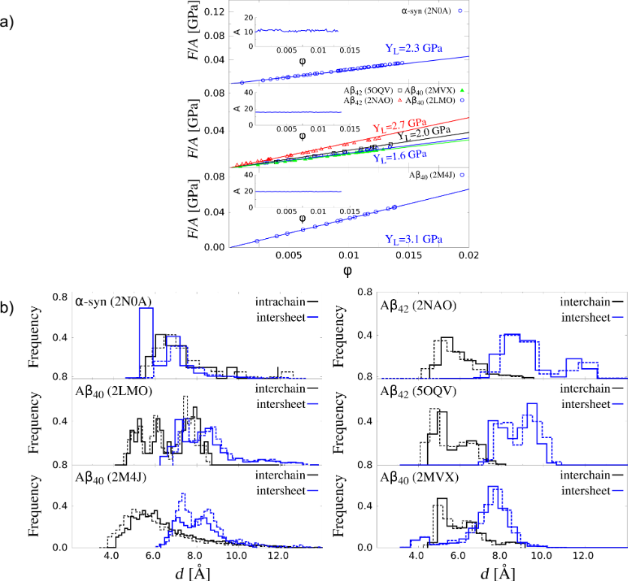Figure 4: Results of tensile deformation. (a) Stress–strain curves of α-synuclein, three Aβ40 and two Aβ42 fibrils. Circles correspond to v = 0.0005 Å/τ. The error bars are the same as the symbol size and they are based on 50 independent simulations for each structure. The insets show the corresponding cross-section areas in nm2 for the corresponding pulling speed. (b) Distributions of HB lengths for (solid lines) and for a finite strain corresponding to the end of the linear regime (dashed lines): for α-syn the final , while for Aβ amyloids. Figure 4: Results of tensile deformation. (a) Stress–strain curves of α-synuclein, three Aβ40 and two Aβ42 fi... Jump to Figure 4

### Shearing deformation

Our results for all systems are presented in Figure 5. The shear deformation for Aβ and α-syn fibrils takes place along the same direction as the tensile deformation (see Figure 3). The initial values of the top-plane areas for each fibril are listed in Table 1. The insets in the left panels of Figure 5 demonstrate that the area A does not change when shear is applied. The values of shear modulus (S) computed for vpull = 0.0005 Å/τ are listed in Table 2. In our studies, these values show a large dependence on the type of Aβ fibril. We find that S for Aβ42 is about 1.6 GPa, while for Aβ40 it is equal to 0.7 GPA. The 2.3-fold increase supports the picture that the Aβ42 fibril is mechanically more stable than the Aβ40. The S value for α-synuclein is comparable to the Aβ40. No experimental data of S for α-synuclein fibril has been reported, but it is expected to be in the range of 1.4–300 MPa. Both limits are typical of microtubules  and collagen  systems, which are assemblies of proteins. Discrepancies between our computational studies and experimental results are expected. One of the sources of divergence is associated with the crystal-like regions that are present in the biological fibrils during each deformation in silico. The initial structure of fibrils is very close to the minimum free energy state (native). Here, the number of hydrogen bonds that participate in the deformation as a whole is larger as reported by all-atom simulations [4,5]. In contrast, during in vitro self-assembly of neurodegenerative fibrils the fibrilization process is dominated by extended regions of amorphous aggregates. Such regions will induce the overall softening of the fibril and therefore a drop in the elastic modulus.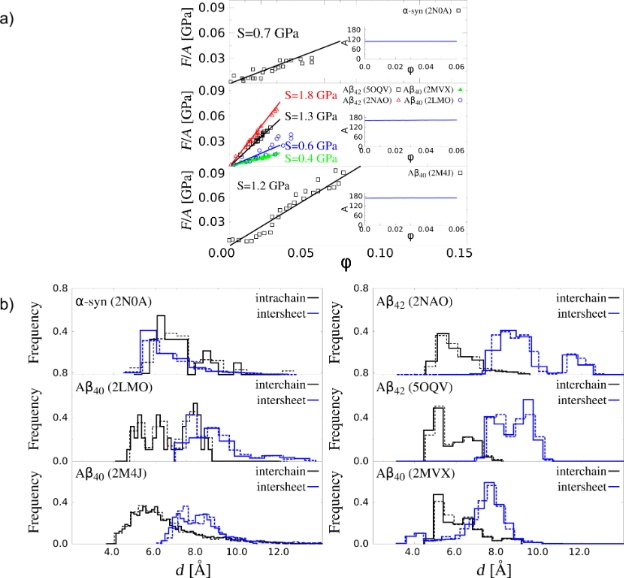Figure 5: Results for shear deformation. (a) Stress–strain curves of α-syn and three Aβ40 and two Aβ42 fibrils. Circles refer to v = 0.0005 Å/τ. The error bars are the same as the symbol size and they are based on 50 independent simulations for each structure. The inset shows the corresponding cross-section area in nm2. (b) Distributions of the HB lengths for (solid lines) and for a finite corresponding to the end of the linear regime (dashed lines), which is 0.04 for α-syn and 0.025 for Aβ amyloids. In the case of Aβ40 with PDB ID: 2M4J has been calculated at strain . Figure 5: Results for shear deformation. (a) Stress–strain curves of α-syn and three Aβ40 and two Aβ42 fibril... Jump to Figure 5

Figure 5b shows the distributions of the characteristic native distances (see Figure 2 for their definition). For β-amyloid and α-synuclein fibrils, the intersheet contacts become slightly stretched, but the distances in the interchain contacts within each sheet are not affected in the case of amyloids. The same analogy can be seen for the intrachain contacts in α-syn fibril. This effect helps the system to keep constant the thickness of the fibril, a condition for the calculation of shear modulus in the linear regime.

### Indentation deformation

Our results for all systems are presented in Figure 6. The indentation deformation for Aβ and α-syn fibrils takes place in the normal direction to the plane z = 0 and at the position L = 0.5L0 (see Figure 3). The initial values of the fibril length for each fibril are listed in Table 1. The values of transversal Young’s modulus (YT) computed for vpull = 0.005 Å/τ are listed in Table 2. In the case of Aβ our results show a large dependency on the type of Aβ fibril. We determine that YT for Aβ42 is about 12 GPa, while for Aβ40 it is equal to 5 GPA. The 2.5-fold increase supports the picture that the Aβ42 fibril is mechanically more stable than Aβ40 . Since Aβ42 aggregates faster than Aβ40  our findings support the correlation between mechanical stability and aggregation propensity as in . The YT value for α-synuclein is comparable to that of Aβ42. The experimental data on YT for α-syn fibril has been reported  and it is by a factor of two smaller than that of Aβ40. Such difference is attributed to an uncontrollable growth of amorphous aggregates during fibrillization that makes the fibril softer. But it is worth mentioning that our theoretical values can be considered as an upper bound in the case of highly ordered fibrils. Moreover, the same result has been observed in all-atom simulation studies .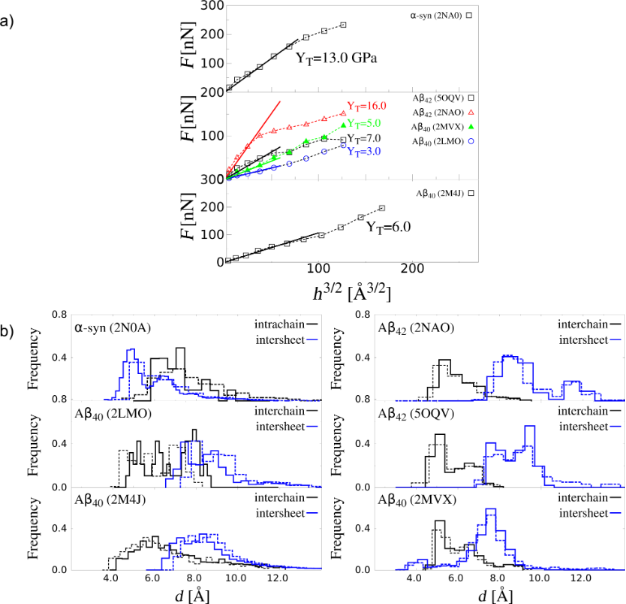Figure 6: Nanoindentation deformation results for different biological fibrils. (a) Force as a function of the indentation depth (h) for α-syn, three Aβ40, and two Aβ42 fibrils. Square symbols refer to vind = 0.005 Å/τ and Rind = 10 nm. The error bars are the same as the symbol size and they are based on 50 independent simulations for each system. The distributions are calculated for h = 0 (solid line) and h = 20 AA in the case of α-syn fibrils and Aβ fibrils (dashed lines). Only in the case of Aβ42 with PDB ID: 2NAO the value h = 9 Å was considered. Figure 6: Nanoindentation deformation results for different biological fibrils. (a) Force as a function of th... Jump to Figure 6

Figure 6b shows the distributions of the characteristic native distances (see Figure 2 for their definition). For Aβ and α-syn fibrils, the intersheet contacts become stretched, but the distances in the interchain contacts within each sheet are shortened in the case of βA fibrils. Analogous effect can be seen for the intrachain contacts in α-syn fibril.

### Thermodynamic characterization of fibrils

Our results regarding the effect of the temperature for each fibril structure are presented in Figure 7. We first study P0 for all fibrils as a function of the temperature. Figure 7a shows that the probability P0 of finding the fibrils in the native state is larger for Aβ40 and Aβ42 than for α-syn at any given temperature. This result is in agreement with a differential calorimetry experiment where it is observed that Tm of β-amyloid fibrils is larger than that of α-syn fibrils [65,66]. In the case of the single fibril, Aβ40 (PDB ID: 2MVX) with two-fold symmetry, it is the most stable at higher temperatures (thermophilic character) among the other two-fold and three-fold β-amyloids. The calibration of our room temperature is 0.35ε/kB. In particular, the folding temperature (Tf) defined in our CG model at P0 equal to 0.5 gives Tf equal to 0.38, 0.42, 0.44, 0.46, and 0.48 in units of ε/kB for the amyloids with the PDB entries 2LMO, 2MJ4, 2NAO, 5OQV, and 2MVX, respectively. With our calibration of ε, the difference between the most (PDB ID: 2MVX) and least (PDB ID: 2LMO) thermophilic fibrils is of the order of 85 °C. Our results indicate that the α-syn fibril is thermally less stable than the Aβ system and this behaviour seems to be intrinsically associated with the extended disordered N-terminus and C-terminus domains. In our model, for α-syn we have determined that Tf is 0.33ε/kB. The difference in temperature with respect to Aβ with PDB IDs 2LMO and 2MVX is 43 °C and 128 °C, respectively. This implies a higher thermodynamic stability of the Aβ systems in comparison with α-syn, which may explain the easier formation of Aβ fibrils over α-syn fibrils. Figure 7b shows thatis larger in the case of α-syn than in the case of Aβ fibrils, at any given T. In addition, Figure 7c presents the RMSF results for all fibrils. We observe that the disordered domains (N- and C-terminus) in α-syn are very flexible in comparison with Aβ fibrils.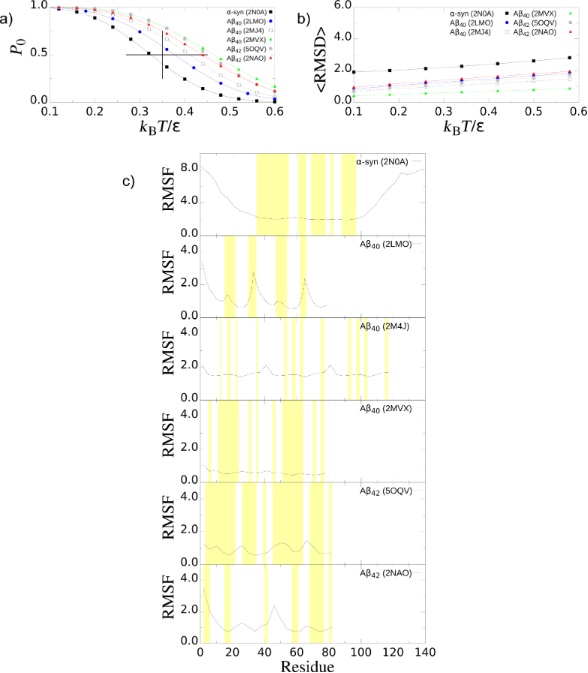Figure 7: Thermodynamic properties of biological fibrils. (a) Probability of finding the fibrils in the native “fibril” state , P0, as a function of the temperature. The vertical line indicates the room temperature equal to 0.35ε/kB and the horizontal line the range of temperatures that offer thermodynamic stability in our model. (b) RMSD of the fibrils. (c) Root-mean-square-fluctuation (RMSF) at room temperature. The β-strand segments in each system are highlighted in yellow. Figure 7: Thermodynamic properties of biological fibrils. (a) Probability of finding the fibrils in the nativ... Jump to Figure 7

## Conclusion

We have carried out molecular dynamics simulations to study the elastic properties of two families of biological fibrils, namely, the β-amyloid and α-synuclein. The elastic properties of this study are the tensile, shear, and indentation deformations. Overall, our results are in agreement with the corresponding experimental values that could be obtained from the literature. Moreover, our method is sensitive to variations in the chain length and the symmetry of the β-amyloid fibril. Our results indicate a higher mechanostability in the case of βA42 fibrils than in the case of Aβ40, namely,,, and. This result is consistent with the results obtained by means of the rupture force . Most importantly, given that the aggregation rate depends on the mechanical stability of the fibrils  our study could provide also hints for the self-assembly of β-amyloid and α-synuclein chains. Our results also indicate an elastic anisotropy namely, YT > YL, for all systems. In the case of α-syn fibrils this difference between YT and YL, is almost one order of magnitude. In contrast, in the case of β-amyloid fibrils the anisotropy is considerably smaller.

We find that this effect is due to the deformation of the hydrophobic core (segments 61–95). We have also confirmed that the large anisotropy in the case of α-syn neither depends on the N-terminus nor the C-terminus domains. Although the mechanical properties indicate some similarity between α-syn and Aβ fibrils, thermodynamic properties reveal differences. That is, β-amyloid fibrils are thermally more stable than α-syn fibrils. β-Amyloid fibrils are, in general, more stable at higher temperatures than at room temperature, while the opposite is the case for α-syn fibrils. In this regard, our method can be used to explore systematically the temperature dependence of the mechanical properties (thermoelasticity) in biological fibrils at experimental length and time scales.

## Acknowledgements

We thank Claudio Perego for critically reading the manuscript. This research has been supported by the National Science Centre, Poland, under grant No. 2015/19/P/ST3/03541, 2015/19/B/ST4/02721, and No. 2017/26/D/NZ1/00466. This project has received funding from the European Union’s Horizon 2020 research and innovation programme under the Marie Skłodowska-Curie grant agreement No. 665778. This research was supported in part by PLGrid Infrastructure. This work was also supported by Department of Science and Technology at Ho Chi Minh city, Vietnam.

#### Interesting articles

##### Nanoscale characterization of the temporary adhesive of the sea urchin Paracentrotus lividus

Ana S. Viana and Romana Santos

##### Liquid-crystalline nanoarchitectures for tissue engineering

Baeckkyoung Sung and Min-Ho Kim

##### Strategy to discover full-length amyloid-beta peptide ligands using high-efficiency microarray technology

Clelia Galati, Natalia Spinella, Lucio Renna, Danilo Milardi, Francesco Attanasio, Michele Francesco Maria Sciacca and Corrado Bongiorno

#### News

##### Beilstein Talk by Marcia C. Barbosa on July 7 – Free registration is open!##### We are looking for an active researcher who is interested in a unique opportunity with a non-profit diamond open access journal.##### The Beilstein Journal of Nanotechnology signed up with the Open Access Switchboard initiativeThis is an Open Access article under the terms of the Creative Commons Attribution License (http://creativecommons.org/licenses/by/4.0). Please note that the reuse, redistribution and reproduction in particular requires that the authors and source are credited.
The license is subject to the Beilstein Journal of Nanotechnology terms and conditions: (https://www.beilstein-journals.org/bjnano)